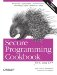# Initializing a Container with Random Numbers

Problem

You want to fill an arbitrary container with random numbers.

Solution

You can use either the generate or generate_n functions from the header with a functor that returns random numbers. See Example 11-13 for an example of how to do this.

Example 11-13. Initializing containers with random numbers

```#include
#include
#include
#include
#include

using namespace std;

struct RndIntGen
{
RndIntGen(int l, int h)
: low(l), high(h)
{ }
int operator( )( ) const {
return low + (rand( ) % ((high - low) + 1));
}
private:
int low;
int high;
};

int main( ) {
srand(static_cast(clock( )));
vector v(5);
generate(v.begin( ), v.end( ), RndIntGen(1, 6));
copy(v.begin( ), v.end( ), ostream_iterator(cout, "
"));
}```

The program in Example 11-13 should produce output similar to:

```3
1
2
6
4```

Discussion

The standard C++ library provides the functions generate and generate_n specifically for filling containers with the result of a generator function. These functions accept a nullary functor (a function pointer or function object with no arguments) whose result is assigned to contiguous values in the container. Sample implementations of the generate and generate_n functions are shown in Example 11-14.

Example 11-14. Sample implementations of generate and generate_n

```template
void generate(Iter_T first, Iter_T last, Fxn_T f) {
while (first != last) *first++ = f( );
}

template
void generate_n(Iter_T first, int n, Fxn_T f) {
for (int i=0; i < n; ++i) *first++ = f( );
}```Secure Programming Cookbook for C and C++: Recipes for Cryptography, Authentication, Input Validation & More
ISBN: 0596003943
EAN: 2147483647
Year: 2006
Pages: 241